Get instant live expert help with Excel or Google Sheets“My Excelchat expert helped me in less than 20 minutes, saving me what would have been 5 hours of work!”

#### Post your problem and you’ll get Expert help in seconds.

Your message must be at least 40 characters
Our professional Expert are available now. Your privacy is guaranteed.

# Change Negative Numbers to Positive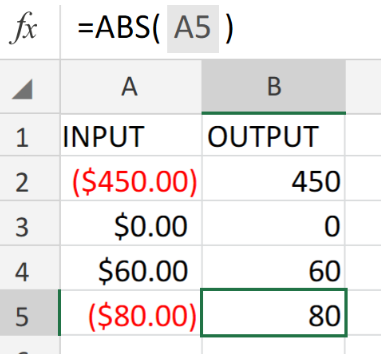Figure 1. of Change Negative Numbers to Positive in Excel.

In order to change negative number values into positive number values, we are going to use the Excel ABS Function.

## Generic Formula

`=ABS(number)`

The number component of the formula syntax us the specific negative number value which we desire to convert to positive.

## How to use the Excel ABS Function

We are going to demonstrate the use of the ABS Function in three simple steps.

1. Enter the number values for conversion into the cells of a column in our worksheet. Be sure to label a separate column for Excel to generate the results. See example illustrated below;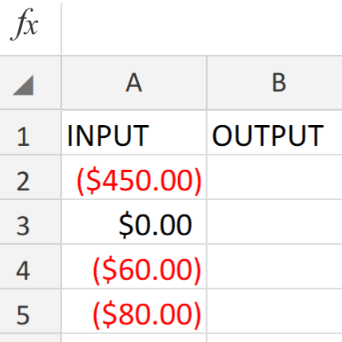Figure 2. of Number Values for Conversion in Excel.

1. Our purpose here is to convert the negative number values in column A above into negative values which Excel will automatically generate into column B.

Hence, the following formula syntax will be entered into the formula bar for cell B2 in our worksheet.

`=ABS(E8)`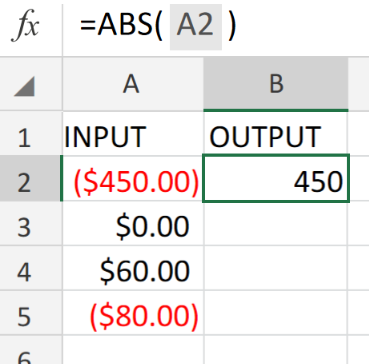Figure 3. of ABS Function in Excel.

1. Modify and copy the formula syntax above into the other cells in the OUTPUT column to achieve the desired results.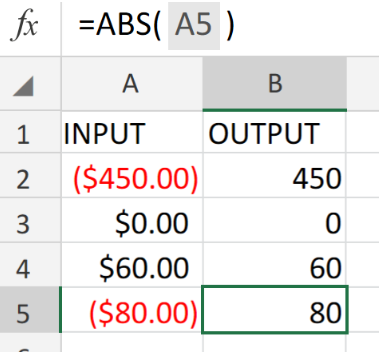Figure 4. of the Excel ABS Function.

## Note

• Negative number values in Excel are usually displayed in the color red.
• The Excel ABS Function is completely automatic. All we have to do is to enter a specific negative number value and the ABS Function will automatically convert to a positive value.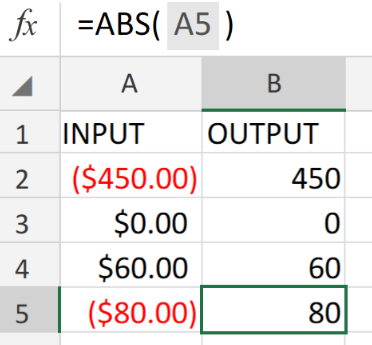Figure 5. of Final Result.

## Instant Connection to an Expert through our Excelchat Service

Our live Excelchat Service is here for you. We have Excel Experts available 24/7 to answer any Excel questions you may have. Guaranteed connection within 30 seconds and a customized solution for you within 20 minutes.

### Did this post not answer your question? Get a solution from connecting with the expert.Another blog reader asked this question today on Excelchat:
Solution exampleshow to transfer data from one worksheet to a new worksheet? If you have a workbook with several TABS (worksheets) is there to save only a given tab?
Solved by E. C. in 19 minsI need an excel formula for my horse racing results spreadsheet. There are four columns - BSP, Low, Win and Lay. The figures for the BSP and Low columns are entered manually. If the figure in the Low column reads 1.01 the formula will take the figure in the BSP column, multiply it by 10, then minus 10 and display it as a minus figure in the Lay column. It will then take the figure in the lay column, deduct 6.5% and then place that in the Win column. Example - BSP is 6, Low is 1.01, then Lay column will read minus 50 and Win column would read 46.75 (50 minus 6.5%),
Solved by K. H. in 13 minsHOW TO USE VLOOKUP FOR ENTIRE SHEETWORK AND MULTIPLE TABS?
Solved by T. Y. in 40 mins## Subscribe to Excelchat.coAnother blog reader asked this question today on Excelchat: